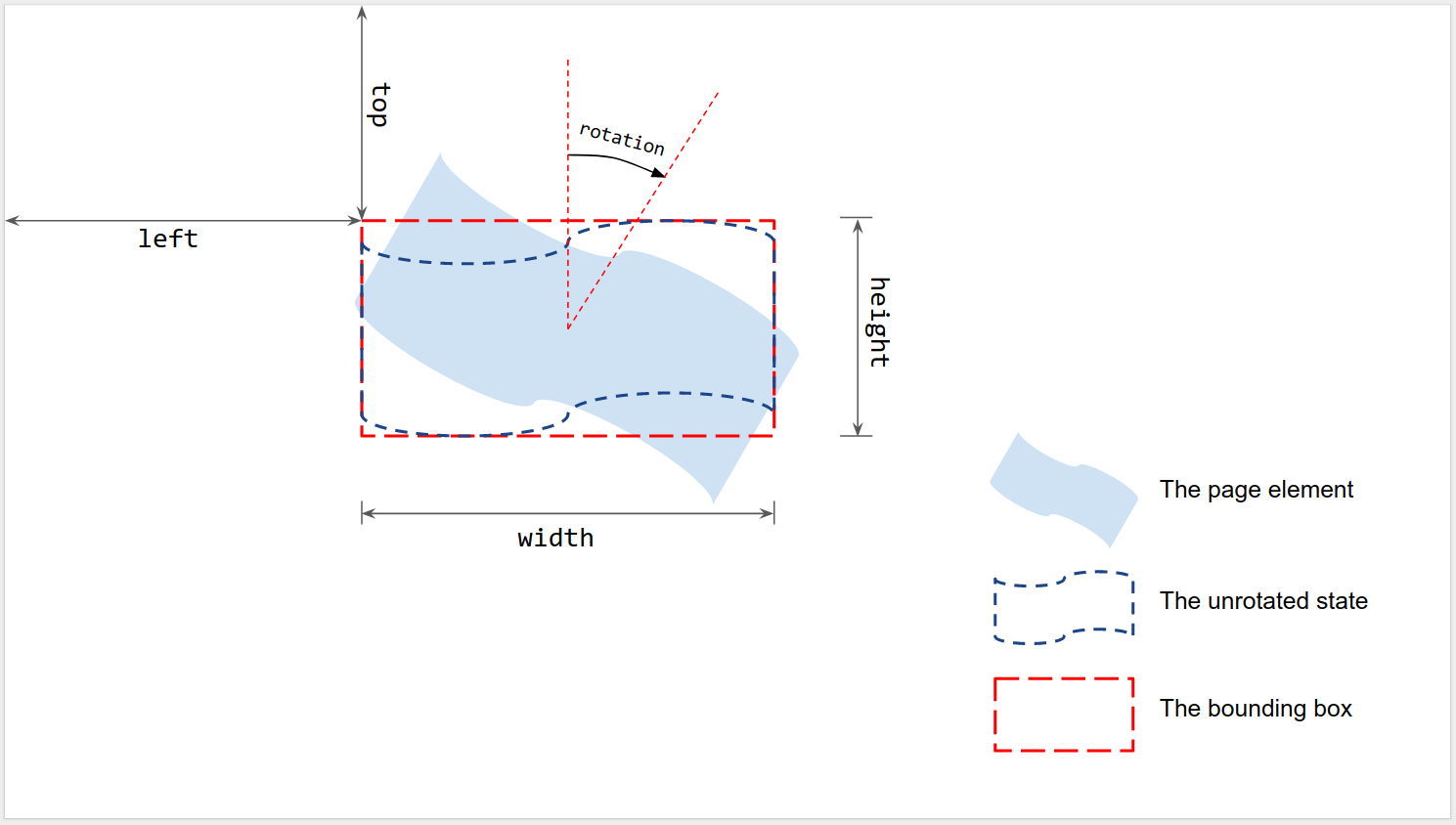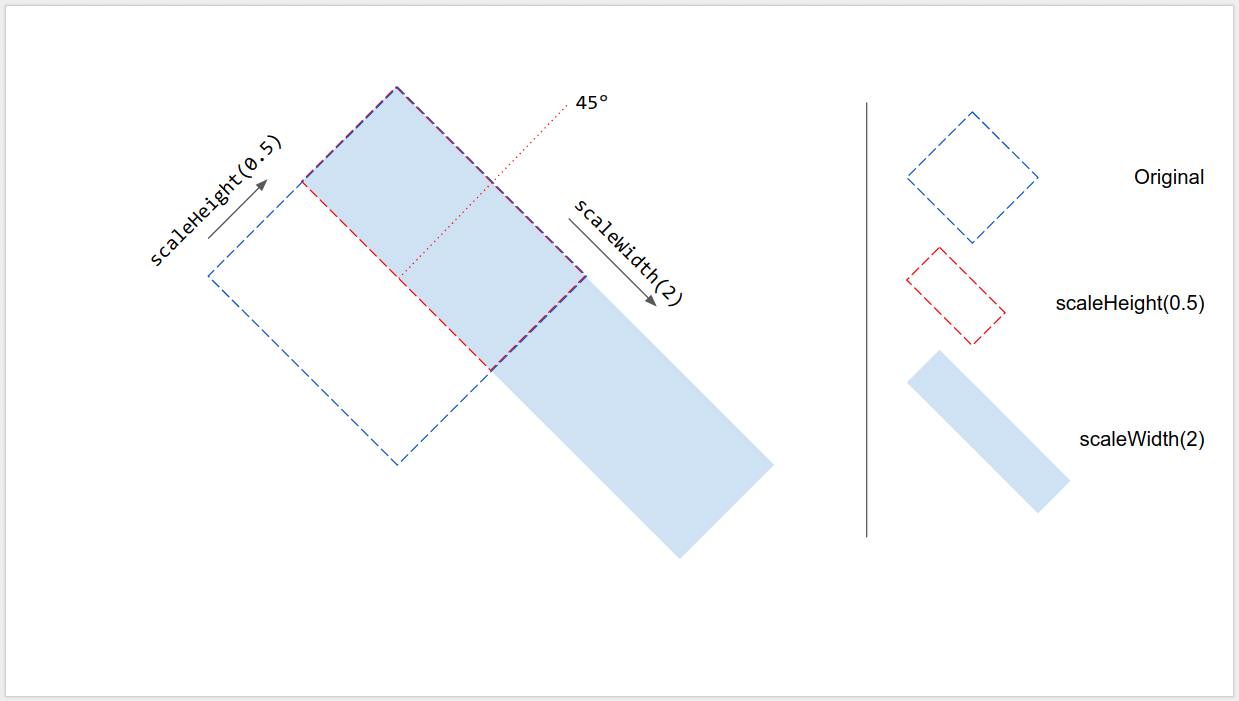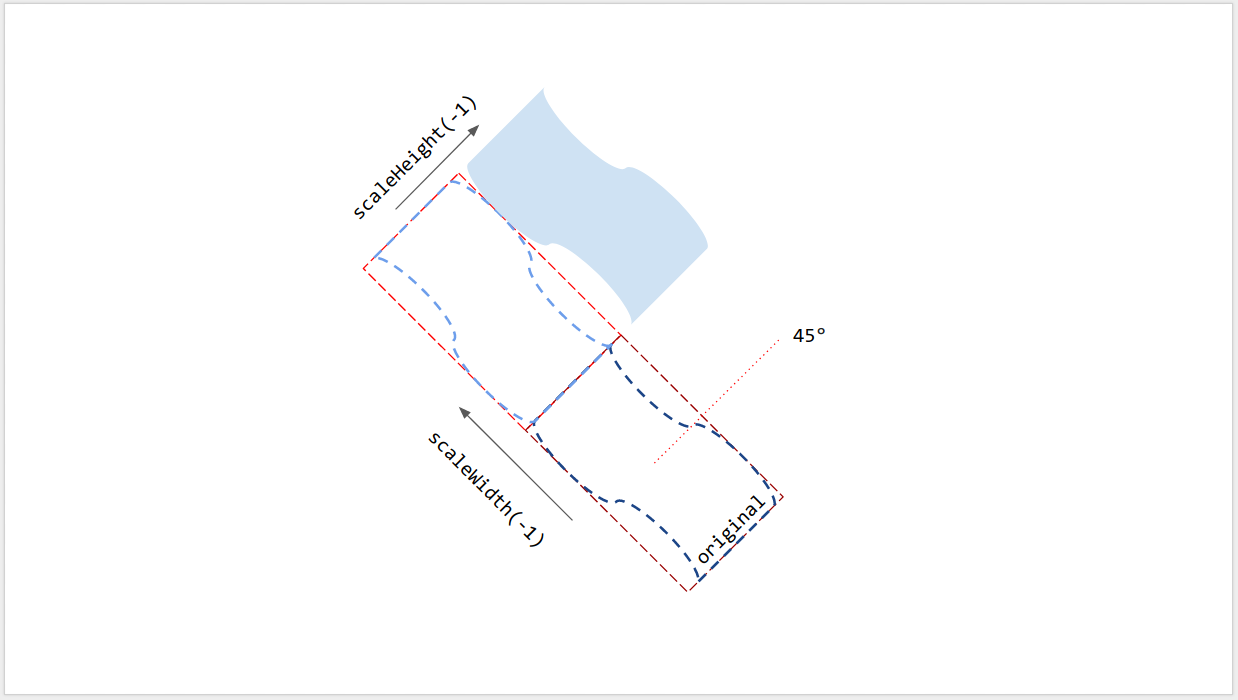# Sizing and positioning page elements

There are two different ways you can get and change the size and position of a page element:

1. Using its getter and setter functions for size and position.
2. Manipulating its affine transform, using its `getTransform()` and `setTransform()` functions while preserving the inherent size.As shown in the figure, size and position are measured with respect to the bounding box of a rendered page element when it has no rotation:

• Left and Top: measured from the upper left corner of the page to the upper left corner of the unrotated bounding box. Use `getLeft()` and `getTop()` to read the values.
• Width and Height: the width and height of the unrotated bounding box. Use `getWidth()` and `getHeight()` to read the values.
• Rotation: the clockwise rotation with respect to the vertical line around the center of the bounding box. Use `getRotation()` to read the value.

All lengths are measured in points (pt). The rotation is measured in degrees (°).

## Setting page element properties

You can set the size and position of a page element when you create it using an insert method such as `insertShape()`. For an existing shape, you can set the size, position, and rotation; you can also set an element's scaling to resize or to reflect it along one of its edges.

### At creation

You can provide position and size information when creating a page element.

``````var slide = SlidesApp.getActivePresentation().getSlides();
var shape = slide.insertShape(SlidesApp.ShapeType.TEXT_BOX, 100, 200, 300, 60);
Logger.log('Left: ' + shape.getLeft() + 'pt; Top: '
+ shape.getTop() + 'pt; Width: '
+ shape.getWidth() + 'pt; Height: '
+ shape.getHeight() + 'pt; Rotation: '
+ shape.getRotation() + ' degrees.');
``````

The above script creates a shape on the first slide of the active presentation with the specified position and size and reads the position and size information of the shape. The expected log is:

``````Left: 100pt; Top: 200pt; Width: 300pt; Height: 60pt; Rotation: 0 degrees.
``````

### Size, position, and rotation

You can update the size and position of a page element after creation:

• Use `setLeft()` and `setTop()` to set the position of the upper left corner of the unrotated bounding box.
• Use `setWidth()` and `setHeight()` to set the rendered width and height of the bounding box.
• Use `setRotation()` to set the clockwise rotation of the bounding box around its center.

The following script creates a shape on the first slide of the active presentation, uses setters to update its position, size, and rotation, and reads the position and size information of the shape.

``````var slide = SlidesApp.getActivePresentation().getSlides();
var shape = slide.insertShape(SlidesApp.ShapeType.RECTANGLE);
shape.setLeft(100).setTop(200).setWidth(50).setHeight(60).setRotation(90);
Logger.log('Left: ' + shape.getLeft()
+ 'pt; Top: ' + shape.getTop()
+ 'pt; Width: ' + shape.getWidth()
+ 'pt; Height: ' + shape.getHeight()
+ 'pt; Rotation: ' + shape.getRotation() + '\u00B0.');
``````

The expected log output from this script is as shown below:

``````Left: 100pt; Top: 200pt; Width: 50pt; Height: 60pt; Rotation: 90°.
``````

The size, position, and rotation setters can be used in any order or combination. Replacing the third line above with the following script will produce the same result:

``````shape.setWidth(55);
shape.setRotation(90).setHeight(60).setLeft(100);
shape.setWidth(50).setTop(200);
``````

### Scaling

Instead of using `setWidth()` and `setHeight()` above to set the size of the shape to an absolute value, `scaleWidth()` and `scaleHeight()` can be used to stretch or squeeze a page element with a relative scaling factor.

``````shape.scaleHeight(0.5).scaleWidth(2);
``````

The figure below depicts how above code works on a 45°-rotated square shape. Note that the upper left corner of the bounding box is fixed during scaling.### Reflection along edge

The argument in `scaleWidth()` and `scaleHeight()` can be negative so that they can be used to flip a page element horizontally or vertically.

``````shape.scaleWidth(-1); // Flip horizontally along the left edge of the bounding box.
shape.scaleHeight(-1); // Flip vertically along the top edge of the bounding box.
``````

The figure below depicts how above code works on a 45°-rotated shape. Note that the page element is flipped along one of the edges of its bounding box but not its center.## Line rotation

Like other page elements, a line's rotation isn't the vertical angle of the line, but the rotation of its bounding box. When you create a line with specified start and end points, its rotation is always 0°. Dragging the endpoints of the line in Google Slides UI changes its vertical angle as well as the size and position of its bounding box, but doesn't change its rotation. Using `setRotation()` rotates the bounding box of the line, which effectively changes its vertical angle. So two lines can have the same visual vertical angle, but different bounding boxes and therefore different size, position, and rotation values.

## Limitations

Some sizing and positioning methods are incompatible with some types of page elements. The table below summarizes the methods which are not compatible with certain types of page elements.

Methods Shape Video Table
getHeight(), getWidth() NO (returns null)
setHeight(), setWidth() NO
setRotation() NO NO
scaleHeight(), scaleWidth() NO

All sizing and positioning methods may give unexpected results if the page element has shearing. All limitations are subject to change. Check reference for up-to-date information.

## Using affine transforms

For advanced control, the size and position of a page element can also be calculated and adjusted through its inherent (native) size and affine transform.

Google Apps script provides similar interface to use affine transform as Google Slides API.

• To read, this article explains the concepts of affine transform and how to infer rendered size from inherent (native) size and transform for page elements. In Apps Script, use
• `getInherentWidth()` and `getInherentHeight()` for the native size of page elements;
• `getTransform()` for the affine transform of the page elements.
• To write, this article describes how to size and position page elements using affine transform to achieve scaling, rotation, reflection, etc. In Apps script, use
• `setTransform()` to set the affine transform of page elements (similar to ABSOLUTE mode);
• `preconcatenateTransform()` to pre-concatenate an affine transform to the current transform of page elements (similar to RELATIVE mode).

The following script creates a shape, sets its transform, reads its inherent size, and reads its affine transform.

``````var slide = SlidesApp.getActivePresentation().getSlides();
var shape = slide.insertShape(SlidesApp.ShapeType.RECTANGLE);
shape.setTransform(SlidesApp.newAffineTransformBuilder()
.setScaleX(2)
.setScaleY(1)
.setTranslateX(100)
.setTranslateY(200)
.build());
Logger.log('Inherent width: ' + shape.getInherentWidth()
+ 'pt; Inherent height: '
+ shape.getInherentHeight() + 'pt.');
``````

The expected log output from this script is as shown below:

``````Inherent width: 236.2pt; Inherent height: 236.2pt.
``````

The resulting shape will have following transform, and rendered size and position:

``````AffineTransform{scaleX=2.0, scaleY=1.0, shearX=0.0, shearY=0.0, translateX=100.0, translateY=200.0}
Left: 100pt; Top: 200pt; Width: 472.4pt; Height: 236.2pt; Rotation: 0°.
``````Need help? Visit our support page.